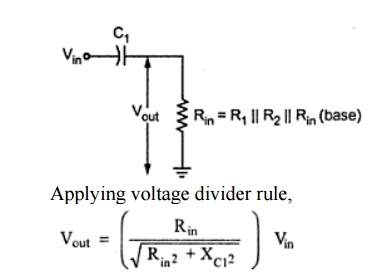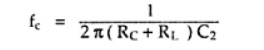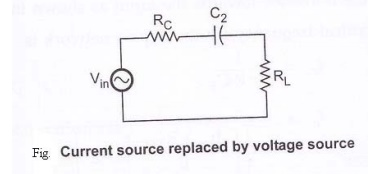Home | | Electronic Circuits I | Low frequency analysis of BJT

# Low frequency analysis of BJT

It has three RC networks that affect its gain as the frequency is reduces below midrange.

Low frequency analysis of BJT:From above figure, it has three RC networks that affect its gain as the frequency is reduces below midrange. These are,

·        RC network formed by the input coupling capacitor C1 and input impedance of the amplifier.

·        RC network formed by the output coupling capacitor C2, resistance looking in at the collector and load resistance.

·        RC network formed by the emitter bypass capacitor CE and resistance looking in at the emitter.

Input RC network:

The following figure shows the input RC network formed by C1 and the input impedance of the amplifier.

The resistance value is Rin = R1 || R2 || Rin(base)A critical point in the amplifier response is generally accepted to occur when the output voltage is 70.7 % of the input. At critical point,At this condition, Rin = Xc1

Overall gain is reduced due to attenuation provided by the input RC network. The reduction in overall gain is given by,The frequency fc at this condition is called lower critical frequency and it is given by,If the resistance of input source is taken into account the above equation becomes,The phase angle in an input RC circuit is expressed asOutput RC network:The above figure shows the output RC network formed by C2, resistance looking in at the collector and load resistance.

The critical frequency for this RC network is given by,The phase angle in output RC network is given as,Bypass network:From above figure,is the resistance looking in at the emitter. It is derived as follows, R= (Vb / βIb) + hie / βWhere RTH = R1 || R2 || Rs. It is the thevenin’s equivalent resistance looking from the base of the transistor towards the input.

The critical frequency for the bypass network isProblem:

Determine the low frequency response of the amplifier circuit shown in the figure.Solution:

It is necessary to analyze each network to determine the critical frequency of the amplifier.The above analysis shows that the input network produces the dominant lower critical frequency. Then the low frequency response of the given amplifier is shown in the following figure.Study Material, Lecturing Notes, Assignment, Reference, Wiki description explanation, brief detail
Electronic Circuits : Frequency Analysis of BJT and MOSFET Amplifiers : Low frequency analysis of BJT |